## The laureates of the annual "Young Mathematician" prize of the St. Petersburg Mathematical Society

Most pictures are clickable

 2022D. D. Cherkashin On extremal combinatorics Summary  |  Texts:        2021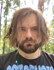A. V. Alpeev On entropy of group actions Summary  |  Texts:            2021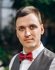N. S. Ustinov Semilineal equations with fractional Laplacians Summary  |  Texts:      2020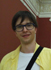N. N. Senik Homogenization of periodic and locally periodic operators Summary  |  Texts:         2019Yu. P. Petrova Exact asymptotics for $L_2$-small finite-dimensional perturbations Summary  |  Texts:      2019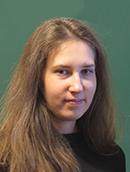M. V. Platonova Evolutional equations and related stochastic processes Summary  |  Texts:          2018M. V. Dolgopolik Constructive nonsmooth analysis and its applications to calculus of variations and control theory Summary  |  Texts:       2017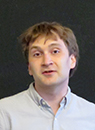A. A. Logunov Geometry of nodal sets of Laplace eigenfunctions Summary  |  Texts:    2015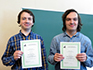P. B. Zatitskiy, D. M. Stolyarov Exact Bellman functions Summary  |  Texts:        2014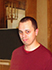S. O. Ivanov Homology of groups and algebras Summary  |  Texts:     2013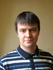K. A. Izyurov Schramm-Loevner evolution (SLE) and correlations in the critical Izing model Summary  |  Texts:      2012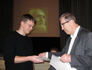A. S. Ananyevsky SL-oriented cohomology theories on algebraic varieties Summary (in Russian)  |  TextsR. S. Pusev Exact asymptotical estimates of small deviations of Gaussian processes in the Hilbert norm Summary (in Russian)  |  Texts 2011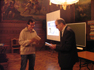Yu. S. Belov Geometry of the de Branges spaces Texts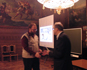F. V. Petrov Asymptotic problems in geometry and combinatorics Texts 2010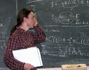P. N. Mnev Discretization of topological quantum field theories Links to the texts 2009A. K. Stavrova Structure of isotropic reductive groups over rings and applications to the theory of anisotropic groups Texts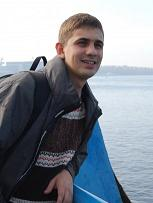S. B. Tikhomirov Works on shadowing for flows Texts 2008A. Yu. Luzgarev Overgroups of exceptional groups Texts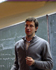V. V. Vysotskii Limit theorems for stochastic models of interacting particles Texts 2007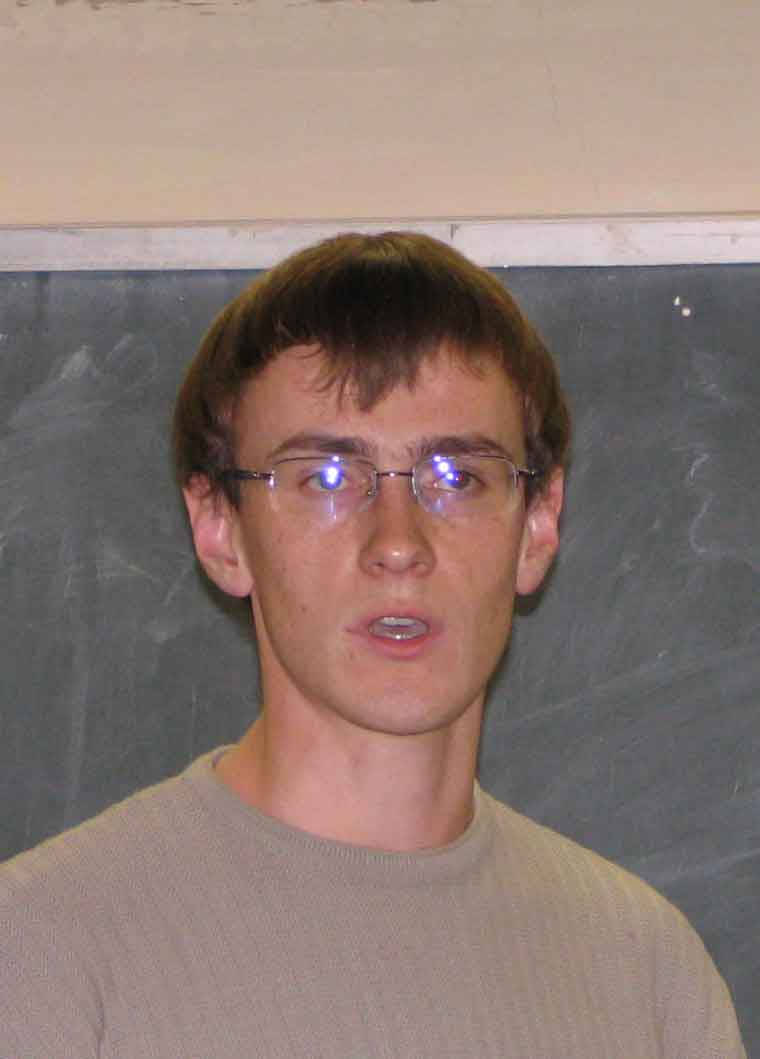K. V. Pervyshev On semantic complexity classes Texts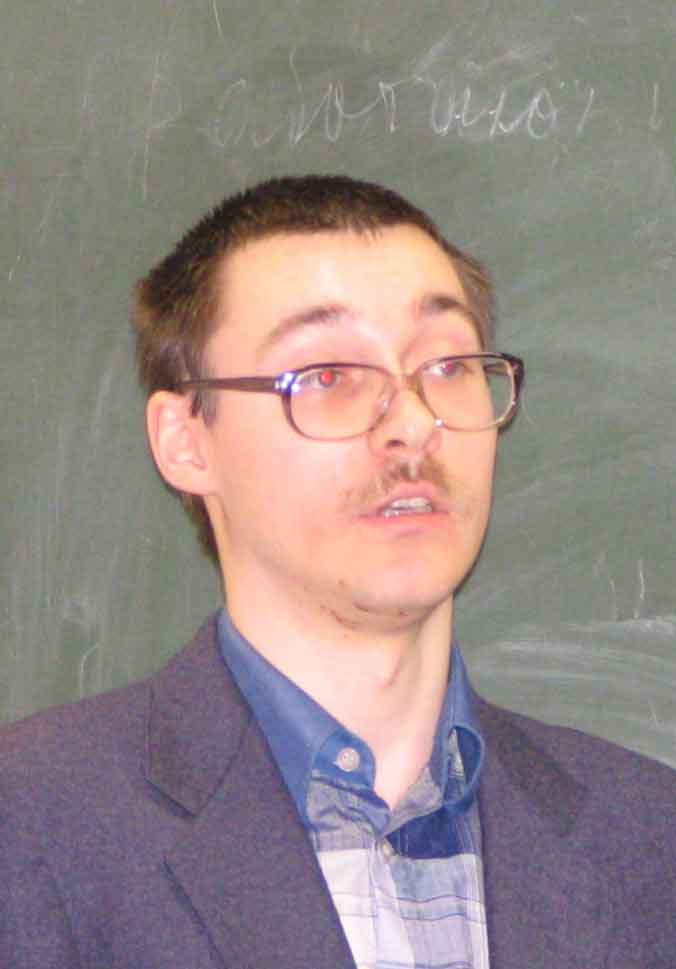V. A. Petrov Motives of the homogenious projective spaces, and applications Texts 2006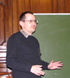N. V. Durov A method of calculation of the Galois group for a polynomial with rational coefficients The text 2005O. A. Tarakanov Shadowing of pseudotrajectories 2004A. D. Baranov Bernstein-type inequalities for model spaces, and their applicationsD. S. Chelkak Inverse problem for a perturbed harmonic oscillator 2003 A. N. Zinoviev Generalized explicit Artin — Hasse and Ivasava formulas 2002 A. G. Ershler Asymptotics of characteristics of random walks on solvable groups 2001 S. G. Kryzhevich Works on the theory of perturbed linear systems of ordinary differential equations A. V. Malyutin Works on braid groups 2000 O. V. Demchenko Arithmetical properties of the Hilbert pairing on formal Honda groups 1999 G. B. Mikhalkin Real algebraic curves, moment map, and amoebas 1998 A. B. Pushnitskii Works on the spectral shift function N. V. Tsilevich Works on Poisson — Dirichlet measures 1996-1997 T. N. Shilkin On regularity of solutions of some problems in mechanics S. K. Smirnov Approximation by vector fields and structure of solenoids O. L. Vinogradov On some exact inequalities in approximation theory 1995 S. V. Ivanov A study of periodic Riemannian metrics 1994 S. M. Shimorin Factorization of analytical functions in Bergmann weight spaces 1993 F. L. Nazarov Uncertainty principle type inequalities in harmonic analysis 1992 I. B. Fesenko Higher theory of class fields D. Yu. Burago Periodic Riemannian metrics 1991 G. Ya. Perel'man Aleksandrov's spaces of curvature bounded from below 1990 A. I. Barvinok Representation theory methods in optimization 1989 A. A. Borichev Convolution equations and an asymptotic holomorphic function O. T. Izhboldin Torsion in Milnor groups 1988 V. A. Kaimanovich Boundaries of random walks on groups N. Yu. Reshetikhin Representation theory for solutions of the Young--Baxter equation 1987 M. Yu. Lyubich Iterations of rational mappings of the Riemann sphere Yu. G. Safarov Works on spectral asymptotics of elliptic operators 1985 M. L. Lifshits A study of the distribution of functionals on trajectories of random processes 1984 V. L. Kobel'skii Models of high-dimensional links D. Yu. Grigor'ev, A. L. Chistov Fast algorithms for polynomial expansions and solutions of algebraic systems 1983 E. K. Sklyanin On some algebraic structures related to the Young--Baxter equation 1982 A. S. Merkur'ev Works on algebraic K-theory V. V. Peller Works on Hankel operators 1981 A. R. Its Finite-gap and isodromic solutions of equations of nonlinear Sroedinger type E. D. Gluskin Works on the geometry of Minkowski spaces 1980 N. E. Barabanov Periodic solutions in control systems N. L. Gordeev Arithmetic problems in the Galois theory O. I. Reinov Approximation $p$-th order property of a Banach space 1978 L. N. Gordeev Constructive models in the classical set theory S. V. Khrushchev Works on analytical functions theory 1977 M. D. Sterlin Estimations of constants in inverse theorems of the constructive function theory A. A. Suslin A solution of J.-P. Serre's problem 1976 E. M. Dyn'kin Works on the properties of a solution of nonhomogeneous Cauchy--Riemann system B. S. Tsirel'son Works on the theory of stochastic differential equations and Banach geometry 1975 O. Ya. Viro Works on topology in codimension two N. A. Shirokov Uniform approximation of functions on closed sets without corner points 1974 Yu. A. Davydov A number of works on limit theorems for Markov random processes 1973 Ya. M. Eliashberg A number of works on global singularity theory 1971 S. A. Vinogradov A number of works on interpolation theorems 1970 Yu. V. Matiyasevich A Diophantic property of recursively enumerable sets 1968 V. V. Zhuk A relation of structure properties of functions and functionals possessing the norm property 1967 V. P. Orevkov Constructive analogues of the Brower theorem 1966 A. S. Blagoveshchenskii Well-defined characteristic problems for an ultra-hyperbolic equation 1965 V. I. Derguzov On the stability of solutions of operator Hamiltonian equations with periodic coefficients A. V. Yakovlev A field embedding problem in the homological algebra setting 1964 V. S. Buslaev A study of short-wave asymptotics of the solution of the diffraction problem on a convex cylinder 1963 B. B. Venkov On the homology of groups of units in division algebras 1962 V. G. Maz'ya Classes of sets and embedding theorems for functional spaces

Back to the main page